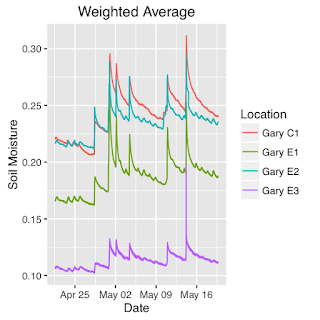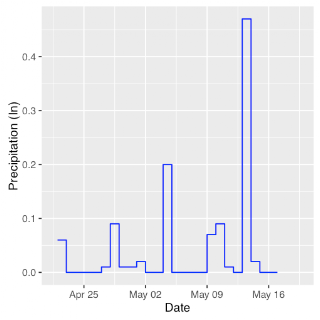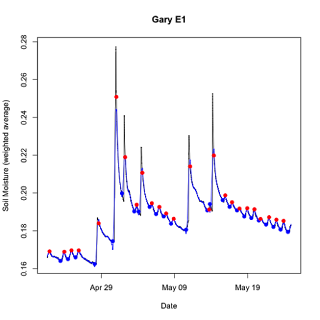## Saturday, May 28, 2016

### What are ways of comparing soil moisture dynamics of rain gardens?

Sandra Albro and I completed installation of monitoring equipment in three experimental parcels and one control parcel in Gary’s Aetna neighborhood on April 19, 2016.  The first month’s monitoring data are now available.  Estimation of depth-weighted soil moisture (m3/m3) is the simplest way to visually compare the dynamics of soil moisture (Figure 1).  The control parcel has the highest water content followed by experimental parcels 2, 1, and 3.  Our weather station in Aetna is temporarily offline, but we can obtain precipitation data from the U.S. Weather Station in Gary (Figure 2).  In general, the spikes in soil moisture correspond to storm events.  Because all of the rain gardens have identical soil mixtures, their variation in water content must reflect differences in local catchment area and the effects of neighboring tree cover.  With predominantly sandy soils, it is not too surprising to observe the rapid decline in soil moisture following precipitation events, but the control parcel (lacking a rain garden) does appear to have a slower rate of decline in soil moisture while maintaining a higher water content.  To move beyond a qualitative comparison of these changes, we will need a quantitative method to find local minima and maxima in a soil moisture time series and determine the slope of soil moisture decline from one local maximum to the next local minimum.  We would also need the method to find the increment of soil moisture from a local minimum to the next local maximum.  Documenting this method and some preliminary results is the purpose of this blog entry.Figure 1.  Patterns of weighted average soil moisture at four sampling locations in Gary.  Parcels are as follows: 1200 Oklahoma (Gary E1), 1035 Oklahoma (Gary E2), 1252 Dakota (Gary E3), and 910 Idaho (Gary C1).Figure 2.  Precipitation patterns for U.S. Weather Service station KGYY in Gary, Indiana.
Finding local extrema (maximum and minimum values) in a time series is the first step in analysis.  Although it would be possible to identify these values visually, a better way is to find a quantitative, rule-based method.  Although I could find no such method in available R libraries, I did find relatively simple algorithm (findpeaks) by Timothée Poisot that uses the diff function in R (https://rtricks.wordpress.com/2009/05/03/an-algorithm-to-find-local-extrema-in-a-vector/).  This algorithm requires specification of a parameter for the analysis interval (with a value between 1 and the length of the entire time series).  I tried a range of values from 0.3% to 25% of the data range and did not find a reasonable match to the observed major spikes and troughs.  Lower parameter values performed better, but the noisy time series tend to produce too many local extrema.  The time series in Figure 1 consist of data reported at 5-minute intervals and thus consists of a mixture signals of process operating at different time scales.

A useful algorithm for smoothing noisy time series is the Loess function in R.  It is an algorithm for fitting local polynomial regressions.  The loess function in R also has a parameter for the size of the sampling interval of the time series.  To smooth out diurnal variations, I set the value of this parameter to a value that equaled the number of samplings per day (288 at 5-minute intervals).  The span parameter for the loess function is thus the ratio of the number of samples per day to the number of days in the time series being analyzed.  With the loess fitted time series, the identification of extrema by the findpeaks function was far less sensitive to values of the sampling interval, and the location of peaks and troughs varied asymptotically with decreasing size of the sampling interval.  I programmed this method in an R script (Slope_Calc.R), and the method correctly identified nearly all of the extrema in the time series for weighted average soil moisture data from the Gary experimental parcel 1 (Figure 3).Figure 3.  Results from the R script (Slope_Calc.R) to find local minima and maxima in the soil moisture time series from the Gary experimental parcel 1.  The black line represents the raw data, the blue line the loess fitted regression, the blue points are the local minima, and the red points are the local maxima.

Once the method estimates local minima and maxima, it then estimates the slope of the observed decline from a local maximum to the next local minimum.  Assuming that the loss of soil moisture is an exponential function, the method applies a linear regression to the log10 values of soil moisture.  The method also calculates the increment in soil moisture from a local minimum to the next local maximum.  Figure 4 shows the resulting slope patterns.Figure 4.  Semi-logarithmic plot of the estimated slopes (red lines) from a linear regression of log transformed of raw data between local maxima and the next local minimum for the weighted average soil moisture data from the Gary experimental parcel 1.  Other lines and points are the same as in Figure 3.

Although the method does correctly estimate the timing of the local maxima and minima, the smoothing functions do have some problems.  On May 13, for example the slope is positive because there was a short interval in which the analysis failed on a smoothing artifact.  The amplitude of the local maximum is also lower than the observed data.  However, the amplitude better matches the loess fitted time series.  Overall, it appears that the method does capture the basic characteristics of the soil moisture dynamics and, thus, appears to be a reasonable method for comparing experimental parcels and controls.  Figure 5 shows an interesting relationship between the magnitude of the soil moisture increment and slope of the subsequent decline in soil moisture.Figure 5.  Plot of slope (1/s) versus soil moisture increment (m3/m3) from the data in Figure 4.  Omitted is the spurious slope from the peak on May 13.  The blue line is the regression line with an r value of -0.82 (p<0.001).
The loss of soil moisture from a rain garden or control plot is a complex function of processes operating at different time scales.  Evapotranspiration and deep infiltration are likely dominant processes in the Aetna neighborhood.  These two process depend on additional factors such as soil temperature, atmospheric relative humidity, vegetation cover and type, and soil porosity.  The results in Figure 5 suggest that it might be possible to find patterns associated with various driving variables in different parts of the time series.  Additionally, the profile of these responses may be useful in comparing water retention by experimental and control plots in different seasons.